# Introduction to Linear Data Structures

Linear Data Structures are a type of data structure in computer science where data elements are arranged sequentially or linearly. Each element has a previous and next adjacent, except for the first and last elements.

## Characteristics of Linear Data Structure:

• Sequential Organization: In linear data structures, data elements are arranged sequentially, one after the other. Each element has a unique predecessor (except for the first element) and a unique successor (except for the last element)
• Order Preservation: The order in which elements are added to the data structure is preserved. This means that the first element added will be the first one to be accessed or removed, and the last element added will be the last one to be accessed or removed.
• Fixed or Dynamic Size: Linear data structures can have either fixed or dynamic sizes. Arrays typically have a fixed size when they are created, while other structures like linked lists, stacks, and queues can dynamically grow or shrink as elements are added or removed.
• Efficient Access: Accessing elements within a linear data structure is typically efficient. For example, arrays offer constant-time access to elements using their index.

Linear data structures are commonly used for organising and manipulating data in a sequential fashion. Some of the most common linear data structures include:

• Arrays: A collection of elements stored in contiguous memory locations.
• Linked Lists: A collection of nodes, each containing an element and a reference to the next node.
• Stacks: A collection of elements with Last-In-First-Out (LIFO) order.
• Queues: A collection of elements with First-In-First-Out (FIFO) order.

## 1. Array

An array is a collection of items of same data type stored at contiguous memory locations.Array

Characteristics of Array Data Structure:

• Homogeneous Elements: All elements within an array must be of the same data type.
• Contiguous Memory Allocation: In most programming languages, elements in an array are stored in contiguous (adjacent) memory locations.
• Zero-Based Indexing: In many programming languages, arrays use zero-based indexing, which means that the first element is accessed with an index of 0, the second with an index of 1, and so on.
• Random Access: Arrays provide constant-time (O(1)) access to elements. This means that regardless of the size of the array, it takes the same amount of time to access any element based on its index.

### Types of arrays:

• One-Dimensional Array: This is the simplest form of an array, which consists of a single row of elements, all of the same data type. Elements in a 1D array are accessed using a single index.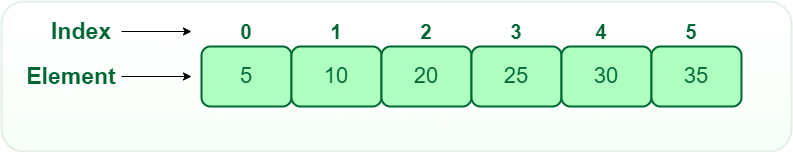One-Dimensional Array

• Two-Dimensional Array: A two-dimensional array, often referred to as a matrix or 2D array, is an array of arrays. It consists of rows and columns, forming a grid-like structure. Elements in a 2D array are accessed using two indices, one for the row and one for the column.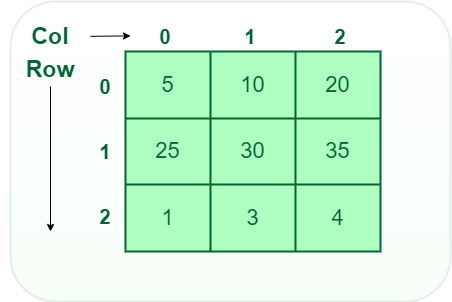Two-Dimensional Array:

• Multi-Dimensional Array: Arrays can have more than two dimensions, leading to multi-dimensional arrays. These are used when data needs to be organized in a multi-dimensional grid.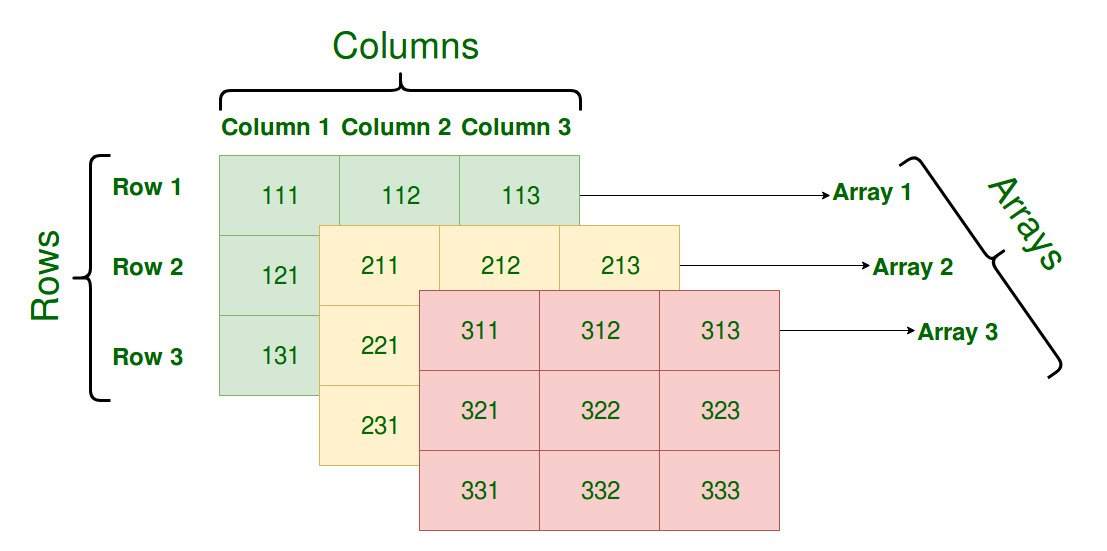Multi-Dimensional Array

### Types of Array operations:

• Accessing Elements: Accessing a specific element in an array by its index is a constant-time operation. It has a time complexity of O(1).
• Insertion: Appending an element to the end of an array is usually a constant-time operation, O(1) but insertion at the beginning or any specific index takes O(n) time because it requires shifting all of the elements.
• Deletion: Same as insertion, deleting the last element is a constant-time operation, O(1) but deletion of element at the beginning or any specific index takes O(n) time because it requires shifting all of the elements.
• Searching: Linear Search takes O(n) time which is useful for unsorted data and Binary Search takes O(logn) time which is useful for sorted data.

A Linked List is a linear data structure which looks like a chain of nodes, where each node contains a data field and a reference(link) to the next node in the list. Unlike Arrays, Linked List elements are not stored at a contiguous location.

### Common Features of Linked List:

• Node: Each element in a linked list is represented by a node, which contains two components:
• Data: The actual data or value associated with the element.
• Next Pointer(or Link): A reference or pointer to the next node in the linked list.
• Head: The first node in a linked list is called the “head.” It serves as the starting point for traversing the list.
• Tail: The last node in a linked list is called the “tail.”

• Singly Linked List: In this type of linked list, every node stores the address or reference of the next node in the list and the last node has the next address or reference as NULL. For example: 1->2->3->4->NULL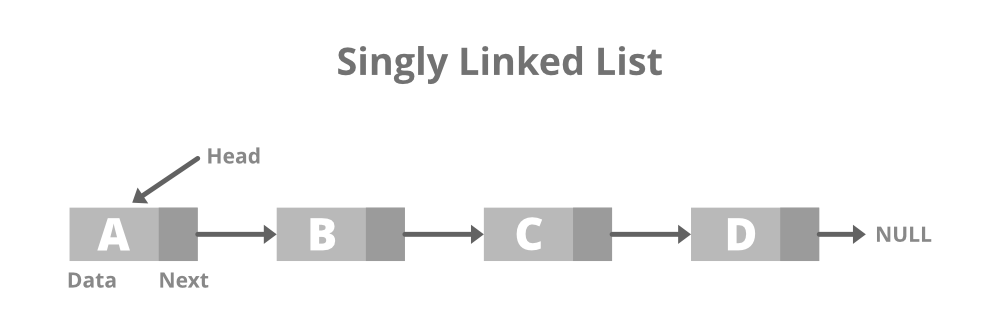• Doubly Linked Lists: In a doubly linked list, each node has two pointers: one pointing to the next node and one pointing to the previous node. This bidirectional structure allows for efficient traversal in both directions.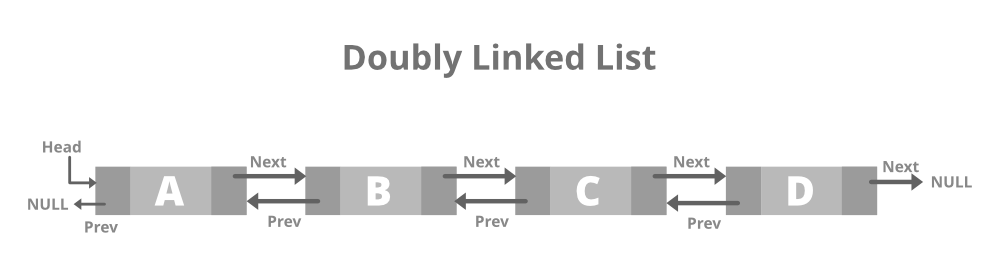• Circular Linked Lists: A circular linked list is a type of linked list in which the first and the last nodes are also connected to each other to form a circle, there is no NULL at the end.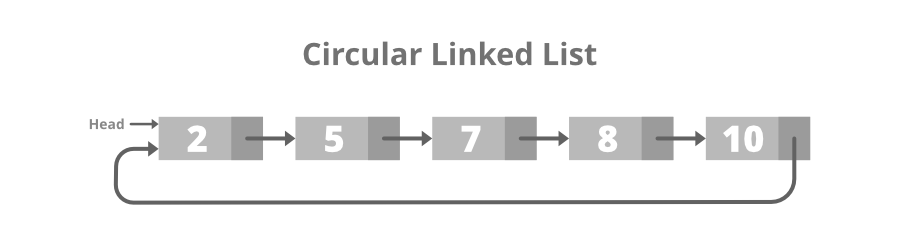### Types of Linked List operations:

• Accessing Elements: Accessing a specific element in a linked list takes O(n) time since nodes are stored in non conitgous locations so random access if not possible.
• Searching: Searching of a node in linked list takes O(n) time as whole list needs to travesed in worst case.
• Insertion: Insertion takes O(1) time if we are at the position where we have to insert an element.
• Deletion: Deletion takes O(1) time if we know the position of the element to be deleted.

## 3. Stack Data Structure

A stack is a linear data structure that follows the Last-In-First-Out (LIFO) principle, meaning that the last element added to the stack is the first one to be removed.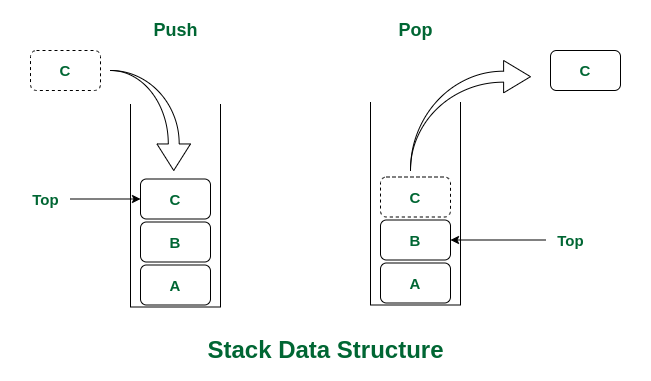Stack Data structure

### Types of Stacks:

• Fixed Size Stack: As the name suggests, a fixed size stack has a fixed size and cannot grow or shrink dynamically. If the stack is full and an attempt is made to add an element to it, an overflow error occurs. If the stack is empty and an attempt is made to remove an element from it, an underflow error occurs.
• Dynamic Size Stack: A dynamic size stack can grow or shrink dynamically. When the stack is full, it automatically increases its size to accommodate the new element, and when the stack is empty, it decreases its size. This type of stack is implemented using a linked list, as it allows for easy resizing of the stack.

### Stack Operations:

• push(): When this operation is performed, an element is inserted into the stack.
• pop(): When this operation is performed, an element is removed from the top of the stack and is returned.
• top(): This operation will return the last inserted element that is at the top without removing it.
• size(): This operation will return the size of the stack i.e. the total number of elements present in the stack.
• isEmpty(): This operation indicates whether the stack is empty or not.

## 4. Queue Data Structure

A queue is a linear data structure that follows the First-In-First-Out (FIFO) principle. In a queue, the first element added is the first one to be removed.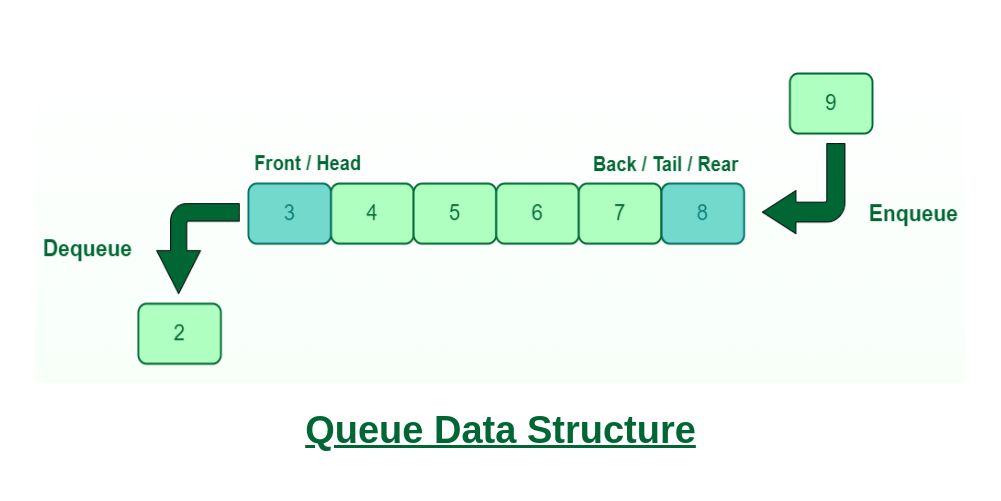Queue Data Structure

### Types of Queue:

• Input Restricted Queue: This is a simple queue. In this type of queue, the input can be taken from only one end but deletion can be done from any of the ends.
• Output Restricted Queue: This is also a simple queue. In this type of queue, the input can be taken from both ends but deletion can be done from only one end.
• Circular Queue: This is a special type of queue where the last position is connected back to the first position. Here also the operations are performed in FIFO order. To know more refer this.
• Double-Ended Queue (Dequeue): In a double-ended queue the insertion and deletion operations, both can be performed from both ends. To know more refer this.
• Priority Queue: A priority queue is a special queue where the elements are accessed based on the priority assigned to them. To know more refer this.

### Queue Operations:

• Enqueue(): Adds (or stores) an element to the end of the queue..
• Dequeue(): Removal of elements from the queue.
• Peek() or front(): Acquires the data element available at the front node of the queue without deleting it.
• rear(): This operation returns the element at the rear end without removing it.
• isFull(): Validates if the queue is full.
• isNull(): Checks if the queue is empty.

## Advantages of Linear Data Structures

• Efficient data access: Elements can be easily accessed by their position in the sequence.
• Dynamic sizing: Linear data structures can dynamically adjust their size as elements are added or removed.
• Ease of implementation: Linear data structures can be easily implemented using arrays or linked lists.
• Versatility: Linear data structures can be used in various applications, such as searching, sorting, and manipulation of data.
• Simple algorithms: Many algorithms used in linear data structures are simple and straightforward.

## Disadvantages of Linear Data Structures

• Limited data access: Accessing elements not stored at the end or the beginning of the sequence can be time-consuming.